(redirected from Electronic smog)
Also found in: Dictionary, Thesaurus, Medical.

energyenergy,
in physics, the ability or capacity to do work or to produce change. Forms of energy include heat, light, sound, electricity, and chemical energy. Energy and work are measured in the same units—foot-pounds, joules, ergs, or some other, depending on the system of
radiated in the form of a wavewave,
in physics, the transfer of energy by the regular vibration, or oscillatory motion, either of some material medium or by the variation in magnitude of the field vectors of an electromagnetic field (see electromagnetic radiation).
as a result of the motion of electric charges. A moving charge gives rise to a magnetic field, and if the motion is changing (accelerated), then the magnetic field varies and in turn produces an electric field. These interacting electric and magnetic fields are at right angles to one another and also to the direction of propagation of the energy. Thus, an electromagnetic wave is a transverse wave. If the direction of the electric field is constant, the wave is said to be polarized (see polarization of lightpolarization of light,
orientation of the vibration pattern of light waves in a singular plane. Characteristics of Polarization

Polarization is a phenomenon peculiar to transverse waves, i.e.
). Electromagnetic radiation does not require a material medium and can travel through a vacuum. The theory of electromagnetic radiation was developed by James Clerk Maxwell and published in 1865. He showed that the speed of propagation of electromagnetic radiation should be identical with that of lightlight,
visible electromagnetic radiation. Of the entire electromagnetic spectrum, the human eye is sensitive to only a tiny part, the part that is called light. The wavelengths of visible light range from about 350 or 400 nm to about 750 or 800 nm.
, about 186,000 mi (300,000 km) per sec. Subsequent experiments by Heinrich Hertz verified Maxwell's prediction through the discovery of radio waves, also known as hertzian waves. Light is a type of electromagnetic radiation, occupying only a small portion of the possible spectrumspectrum,
arrangement or display of light or other form of radiation separated according to wavelength, frequency, energy, or some other property. Beams of charged particles can be separated into a spectrum according to mass in a mass spectrometer (see mass spectrograph).
of this energy. The various types of electromagnetic radiation differ only in wavelength and frequency; they are alike in all other respects. The possible sources of electromagnetic radiation are directly related to wavelength: long radio waves are produced by large antennas such as those used by broadcasting stations; much shorter visible light waves are produced by the motions of charges within atomsatom
[Gr.,=uncuttable (indivisible)], basic unit of matter; more properly, the smallest unit of a chemical element having the properties of that element. Structure of the Atom
; the shortest waves, those of gamma radiationgamma radiation,
high-energy photons emitted as one of the three types of radiation resulting from natural radioactivity. It is the most energetic form of electromagnetic radiation, with a very short wavelength (high frequency).
, result from changes within the nucleusnucleus,
in physics, the extremely dense central core of an atom. The Nature of the Nucleus
Composition

Atomic nuclei are composed of two types of particles, protons and neutrons, which are collectively known as nucleons.
of the atom. In order of decreasing wavelength and increasing frequency, various types of electromagnetic radiation include: electric waves, radioradio,
transmission or reception of electromagnetic radiation in the radio frequency range. The term is commonly applied also to the equipment used, especially to the radio receiver.
waves (including AM, FM, TV, and shortwaves), microwaves, infrared radiationinfrared radiation,
electromagnetic radiation having a wavelength in the range from c.75 × 10−6 cm to c.100,000 × 10−6 cm (0.000075–0.1 cm).
invisible electromagnetic radiation between visible violet light and X rays; it ranges in wavelength from about 400 to 4 nanometers and in frequency from about 1015 to 1017 hertz.
, X raysX ray,
invisible, highly penetrating electromagnetic radiation of much shorter wavelength (higher frequency) than visible light. The wavelength range for X rays is from about 10−8 m to about 10−11
, and gamma radiation. According to the quantum theoryquantum theory,
modern physical theory concerned with the emission and absorption of energy by matter and with the motion of material particles; the quantum theory and the theory of relativity together form the theoretical basis of modern physics.
, light and other forms of electromagnetic radiation may at times exhibit properties like those of particles in their interaction with matter. (Conversely, particles sometimes exhibit wavelike properties.) The individual quantum of electromagnetic radiation is known as the photonphoton
, the particle composing light and other forms of electromagnetic radiation, sometimes called light quantum. The photon has no charge and no mass. About the beginning of the 20th cent.
and is symbolized by the Greek letter gamma. Quantum effects are most pronounced for the higher frequencies, such as gamma rays, and are usually negligible for radio waves at the long-wavelength, low-frequency end of the spectrum.

Energy transmitted through space or through a material medium in the form of electromagnetic waves. The term can also refer to the emission and propagation of such energy. Whenever an electric charge oscillates or is accelerated, a disturbance characterized by the existence of electric and magnetic fields propagates outward from it. This disturbance is called an electromagnetic wave. The frequency range of such waves is tremendous, as is shown by the electromagnetic spectrum in the table. The sources given are typical, but not mutually exclusive.

In theory, any electromagnetic radiation can be detected by its heating effect. This method has actually been used over the range from x-rays to radio. lonization effects measured by cloud chambers, photographic emulsions, ionization chambers, and Geiger counters have been used in the γ- and x-ray regions. Direct photography can be used from the γ-ray to the infrared region.

Electromagnetic spectrum
Frequency, Wavelength,
Hz m Nomenclature Typical source
1023 3 × 10-15 Cosmic photons Astronomical
1022 3 × 10-14 γ-rays Radioactive nuclei
1021 3 × 10-13 γ-rays, x-rays
1020 3 × 10-12 x-rays Atomic inner shell
Positron-electron annihilation
1019 3 × 10-11 Soft x-rays Electron impact on a solid
1018 3 × 10-10 Ultraviolet, x-rays Atoms in sparks
1017 3 × 10-9 Ultraviolet Atoms in sparks and arcs
1016 3 × 10-8 Ultraviolet Atoms in sparks and arcs
1015 3 × 10-7 Visible spectrum Atoms, hot bodies,
molecules
1014 3 × 10-6 Infrared Hot bodies, molecules
1013 3 × 10-5 Infrared Hot bodies, molecules
1012 3 × 10-4 Far-infrared Hot bodies, molecules
1011 3 × 10-3 Microwaves Electronic devices
1010 3 × 10-2 Microwaves, radar Electronic devices
109 3 × 10-1 Radar Electronic devices
Interstellar hydrogen
108 3 Television, FM radio Electronic devices
107 30 Short-wave radio Electronic devices
106 300 AM radio Electronic devices
105 3000 Long-wave radio Electronic devices
104 3 × 104 Induction heating Electronic devices
103 3 × 105 Electronic devices
100 3 × 106 Power Rotating machinery
10 3 × 107 Power Rotating machinery
1 3 × 108 Commutated direct current
0 Infinity Direct current Batteries

Fluorescence is effective in the x-ray and ultraviolet ranges. Bolometers, thermocouples, and other heat-measuring devices are used chiefly in the infrared and microwave regions. Crystal detectors, vacuum tubes, and transistors cover the microwave and radio frequency ranges. See Diffraction, Electromagnetic wave, Gamma rays, Heat radiation, Infrared radiation, Interference of waves, Light, Maxwell's equations, Polarization of waves, Reflection of electromagnetic radiation, Refraction of waves, Scattering of electromagnetic radiation, Ultraviolet radiation, Wave motion, X-rays

A disturbance that can travel through a vacuum as well as through a material medium, light and radio waves being familiar forms. It consists of oscillating (time-varying) electric and magnetic fields with directions at right angles to each other and to the direction of propagation. The two fields are bound together, the time-varying electric and magnetic components regenerating each other in an endless cycle that moves from one point to the next through space. The radiation transfers energy and also momentum. It travels through a vacuum at the speed of light, c , which is a fundamental constant equal to about 3 × 105 km s–1. The speed is slightly reduced on entering a medium, such as air or glass.

Electromagnetic radiation is caused by the acceleration of charged particles, such as electrons. Its propagation through space can be fully described in terms of wave motion. Like other periodic waves, electromagnetic waves have a wavelength λ and a frequency ν, which are related by λν = c . Reflection, refraction, interference, and polarization can be explained in terms of wave motion. When radiation interacts with matter, however, it exhibits particle-like behavior, as when it undergoes absorption or emission. It thus has a dual wave–particle nature. A particulate nature was originally proposed by Newton but in its present form the concept is part of quantum theory. Light and other kinds of radiation interact with matter as quanta. A quantum of radiation of frequency ν transfers energy h ν and momentum h ν/c 2, where h is the Planck constant. The quantum of electromagnetic radiation is the photon. Light, etc., is thus absorbed by or emitted from atoms or molecules in the form of photons.

The range of frequencies (or wavelengths) of electromagnetic radiation is known as the electromagnetic spectrum (see illustration). The spectrum can be divided into various regions, which are not sharply delineated. These regions range from low-frequency low-energy radio waves through infrared radiation, light, ultraviolet radiation, X-rays, to high-frequency high-energy gamma rays. Most astronomical observations measure some form of electromagnetic radiation.

Classical radiation theory (Maxwell’s theory). The physical reasons for the existence of a free electromagnetic field (that is, a self-sustaining field that has become independent of the sources that induced it) are closely associated with the fact that electromagnetic waves propagate from sources—charges and currents —with a finite speed c (in a vacuum c˜3 X 1010 cm/sec) rather than instantaneously. If the radiation source (such as an alternating current) disappears at a certain instant, the field will not disappear instantaneously in the entire space: at points remote from the source it will disappear only after a finite time interval. It follows from Maxwell’s theory that a change in an electric field E in time gives rise to a magnetic field H, and the change in H gives rise to a vortex electric field. Hence it follows that only a variable electromagnetic field, in which both components—E and H—constantly excite each other by changing continuously, may be self-sustaining.

In the process of radiation an electromagnetic field carries energy away from the source. The energy flux density of the field (the quantity of energy per unit time passing through a unit area oriented perpendicular to the direction of the flux) is determined by the Poynting vector P, which is proportional to the vector product [EH].

The radiation intensity Eradis the energy removed from the source by the field per unit time. Its order of magnitude can be estimated by calculating the product of the area of the closed surface encompassing the source and the average value of the absolute flux density P on this surface (P˜EH). The surface is usually selected in the form of a sphere of radius R (its area is on the order of R2), and Erad is calculated from the limit R → ∞:

(1) radR2ǀEHǀR → ∞

(E and H are the absolute values of the vectors E and H).

In order that this quantity does not disappear—that is, in order for a free electromagnetic field to exist far from the source —both E and H must not decrease more rapidly than \/R. This requirement is satisfied if moving charges under acceleration are the field sources. Near the charges the fields are Coulomb fields proportional to l/R2, but at large distances non-Coulomb fields Eand H, which attenuate as l/R, begin to play the primary role.

RADIATION OF A MOVING CHARGE. A point charge is the simplest field source. A resting charge has no radiation; nor may a uniformly moving charge (in a vacuum) be a source of radiation. However, a charge moving under acceleration does radiate. Direct calculations based on Maxwell’s equations show that the intensity of its radiation is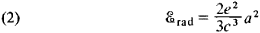where e is the magnitude of the charge and a is its acceleration. (Here and below the Gaussian system of units is used.) Depending on the physical nature of the acceleration the radiation is sometimes given special names. For example, the radiation that arises during the deceleration of charged particles in matter as a result of the action of the Coulomb fields of automic nuclei and electrons is called bremsstrahlung. The radiation of a charged particle moving in a magnetic field that deflects its trajectory is called synchrotron radiation. It is observed, for example, in cyclic charged-particle accelerators.

In a particular case, when a charge undergoes a harmonic oscillation, the acceleration a is equal in magnitude to the product of the deviation of the charge from a state of equilibrium (x = x0 sin ωt, where x0 is the amplitude of the deviation of x) and the square of the frequency s. The radiation intensity, averaged with respect to time t, increases very rapidly (proportional to ω4) as the frequency increases: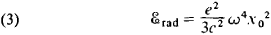ELECTRIC DIPOLE RADIATION. Two interconnected, co-oscillating opposite charges that are equal in magnitude are the simplest system that may serve as a source of radiation. They form a dipole with a variable moment. For example, if the charges of the dipole oscillate harmonically against each other, then the electric dipole moment changes according to the law d = d0 sin wt (ω is the frequency of oscillations, and do is the amplitude of moment d). The radiation intensity of such a dipole averaged with respect to the time t is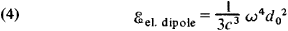The radiation emanating from an oscillating dipole is anisotropic—that is, the energy emitted in various directions is not identical. Radiation is entirely absent along the axis of oscillations and is at a maximum at right angles to the axis of oscillations. For all intermediate directions the angular distribution of the radiation changes in proportion to sin2θ, where the angle θ is read from the direction of the axis of oscillations. If the direction of the axis of oscillations of a dipole changes over time, then the average angular distribution becomes more complex.

Real radiators usually include a set of charges. Precise consideration for all details of the motion of each charge in the study of radiation is unnecessary and often impossible. Indeed, radiation is defined by the values of the fields far from the source— that is, where the details of the distribution of the charges and currents in the radiator have a slight effect. This makes it possible to replace the true distribution of the charges with an approximate distribution. Consideration of a radiating system as a single charge, equal in magnitude to the sum of the charges of the system, is the crudest, “zero-order” approximation. In an electrically neutral system, the sum of whose charges is equal to zero, radiation is absent in this approximation. In the next approximation (the first), the positive and negative charges of the system “tend” imaginarily toward the centers of their distribution. For an electrically neutral system this means conceptual replacement by an electric dipole radiating according to (4). This approximation is called a dipole approximation, and the corresponding radiation is called electric dipole radiation.

ELECTRIC QUADRUPOLE AND HIGHER MULTIPOLE RADIATION. If a system of charges has no dipole radiation—for example, because the dipole moment is equal to zero—then it is necessary to take into account the next approximation, in which the system of charges (the radiation source) is regarded as a quadrupole. The simplest quadrupole is two dipoles that have moments of equal magnitude and opposite direction. An even more detailed description of a radiating system of charges is given by consideration of subsequent approximations, in which the charge distribution is described by multipoles of higher orders (a dipole is called a first-order multipole, a quadrupole a second-order multipole, and so on).

It is important to note that in each successive approximation the radiation intensity is approximately (c/v)2 times less than in the previous one (if, of course, it is not absent for some reason). If the radiator is nonrelativistic—that is, if all charges have velocities much less than the speed of light (v/c « 1)—then the lower, nonvanishing approximation plays the main role. Thus, if dipole radiation is present it is the primary radiation, and all other higher multipole corrections are extremely small and need not be taken into account. In the case of the radiation of relativistic particles, however, the description of radiation by means of multipoles becomes ineffective, since the contribution of higher-order multipoles ceases to be small.

MAGNETIC DIPOLE RADIATION. In addition to electric dipoles and higher multipoles, magnetic dipoles and multipoles may also be radiation sources (magnetic dipole radiation is generally the primary radiation). The distribution pattern of a magnetic field at a great distance from the circuit through which the current that gives rise to this field is flowing is similar to the distribution pattern of an electric field far from an electric dipole. The analogue of the electric dipole moment, the dipole magnetic moment M, is determined by the current strength I in the circuit and by the circuit’s geometry. For a plane circuit the absolute magnitude of the moment is M = (e/c )IS, where S is the area within the circuit. The formulas for the intensity of magnetic dipole radiation are almost the same as for electric radiation, except that they contain the magnetic moment M instead of the electric dipole moment d. Thus, if the magnetic moment changes according to the harmonic law M = M0 sin cat (for this the current strength I in the circuit must change harmonically), the radiation intensity averaged with respect to time is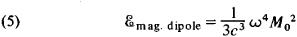Here M0 is the amplitude of the magnetic moment M.

The ratio of the magnetic and electric dipole moments is of the order of v/c, where v is the rate of motion of the charges forming the current; from this it follows that the intensity of magnetic dipole radiation is (c/v)2 times less than that of electric dipole radiation if, of course, the latter is present. Thus, the intensities of magnetic dipole and electric quadrupole radiation are of the same order of magnitude.

RADIATION OF RELATIVISTIC PARTICLES. The synchrotron radiation of charged particles in circular (ring) accelerators is one of the most important examples of radiation of relativistic particles. A sharp difference from nonrelativistic radiation is manifested here in the spectral composition of the radiation: if the revolution frequency of a charged particle in an accelerator is equal to ω (a nonrelativistic radiator would emit waves of the same frequency), then its radiation intensity has a maximum at a frequency ωmax ˜ ³3ω, where ³ = [1 — (v/c)2]-1/2; that is, when vc most of the radiation is found at frequencies higher than ω. Such radiation is directed nearly at a tangent to the orbit of the particle, mainly in the direction of its motion.

An ultrarelativistic particle may radiate electromagnetic waves even if it is moving in a straight line and uniformly (but only in matter, not in a vacuum!). This radiation, called Cherenkov radiation, arises if the velocity of a charged particle in a medium exceeds the phase velocity of light in this medium (uPhase = c/n, where n is the index of refraction of the medium). The radiation appears because the particle “overtakes” and breaks away from the field that gives rise to it.

Quantum radiation theory. It is has already been stated above that classical theory gives only an approximate description of radiation processes (the entire physical world is in principle a “quantum” world). However, physical systems also exist whose radiation cannot be described even approximately in conformance with experiments from the standpoint of classical theory. An important feature of such quantum systems as the atom or molecule is that their internal energy does not change continuously but may assume only certain values, forming a discrete set. The transition of a system from a state with one energy to a state with another takes place in a stepwise manner; because of the law of conservation of energy, upon such a transition a system must lose or acquire a certain “portion” of energy. This process is realized most often in the form of emission or absorption of a quantum of radiation—a photon—by the system. The energy of a quantum is Є³ =ħω where ħ is Planck’s constant (ħ = 1.05450 X 10-27 erg/sec), and ω is the angular frequency. A photon always acts as a unified whole, is emitted and absorbed “in its entirety,” in a single event, and has a definite energy, momentum, and spin (the projection of the angular momentum on the direction of the momentum)—that is, it has a number of corpuscular properties. At the same time the photon differs sharply from conventional classical particles in that it also has wave features. This dual character of the photon is a particular phenomenon of particle-wave dualism.

Quantum electrodynamics is a consistent quantum theory of radiation. However, many results that bear on the processes of the radiation of quantum systems can be obtained from the simpler semiclassical radiation theory. The formulas of the latter, according to the correspondence principle, should, within certain limits, give the results of classical theory for some limiting transition. Thus, a profound analogy is established between the quantities that characterize radiation processes in quantum and classical theories.

ATOMIC RADIATION. A system consisting of a nucleus and an electron moving in its Coulomb field must be in one of the discrete states (at a certain energy level). All states except the ground state (the state with the lowest energy) are unstable. An atom in an unstable (excited) state, even if isolated, passes into a state of lower energy. This quantum transition is accompanied by the emission of a photon; such radiation is called spontaneous radiation. The energy removed by the photon, Єϒ = ħω, is equal to the difference between the energies of the initial state and the final state j of the atom (ЄiЄj; Єi—Єj); the formula of N. Bohr for radiation frequencies follows from this: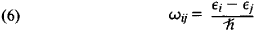It is important to note that such characteristics of spontaneous radiation as the direction of propagation (for a set of atoms, the angular distribution of their spontaneous radiation) and polarization do not depend on the radiation of other objects, such as an external electromagnetic field.

Bohr’s formula (6) defines the discrete set of frequencies, and consequently the wavelengths, of atomic radiation. It explains why the spectra of atomic radiation have the well-known “line” character—each line of the spectrum corresponds to one of the quantum transitions of the atoms of the particular substance.

RADIATION INTENSITY. In quantum theory, as in classical theory, electric dipole and higher multipole radiation may be examined. If a radiator is nonrelativistic, the primary radiation is the electric dipole radiation whose intensity is determined by a formula close to the classical formula: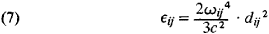The quantities dij, which are a quantum analogue of the electric dipole moment, are found to be nonzero only when certain relations exist between the quantum numbers of the initial state /’ and the final state j (the selection rules for dipole radiation). Quantum transitions that satisfy such selection rules are called allowed transitions (the allowed electric dipole radiation is actually meant). By contrast, transitions of higher multipole levels are said to be forbidden. This prohibition is relative: forbidden transitions have a relatively low probability—that is, the radiation intensity corresponding to them is small. The states from which transitions are “forbidden” are comparatively stable (long-lived); they are called metastable states.

Quantum radiation theory makes possible an explanation not only of the difference in intensity of various lines but also of the intensity distribution within each line, and in particular the width of spectral lines.

Not only atoms but also more complex quantum systems may be sources of electromagnetic radiation. The general methods for describing the radiation of such systems are the same as those used in the study of atoms, but the specific features of the radiation are extremely diverse. For example, molecular radiation has more complex spectra than does atomic radiation. High energy of individual quanta (³-quanta) and comparatively low radiation intensity are typical of the radiation of atomic nuclei.

Electromagnetic radiation frequently also arises during mutual transformations of elementary particles (such as the annihilation of electrons and positrons and the decay of the neutral pi-meson).

REFERENCES

Tamm, I.E. Osnovy teorii elektrichestva, 7th ed. Moscow, 1957.
Ivanenko, D., and A. Sokolov. Klassicheskaia teoriia polia. Moscow-Leningrad, 1949.
Ivanenko, D., and A. Sokolov. Kvantovaia teoriia polia. Moscow-Leningrad, 1952.
Akhiezer, A. I., and V.B. Berestetskii. Kvantovaia elektrodinamika, 2nd ed. Moscow, 1959.
Landau, L. D., and E.M. Lifshits. Teoriia polia, 5th ed. Moscow, 1967. (Teoreticheskaia fizika, vol. 2.)

V. I. GRIGOR’EV